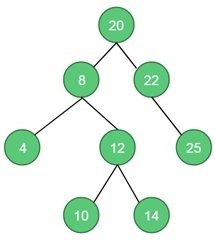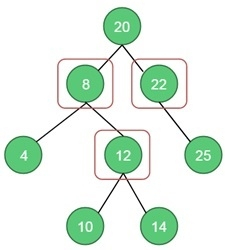# Vertex cover Problem

For an undirected graph, the vertex cover is a subset of the vertices, where for every edge (u, v) of the graph either u or v is in the set.

Using a binary tree, we can easily solve the vertex cover problem.

This problem can be divided into two sub-problems. When the root is part of the vertex cover. For this case, the root covers all children edges. We can simply find the size of vertex cover for left and right sub-tree, and add 1 for the root.

## Input and Output

Input:
A binary tree.Output:
The vertex cover is 3.#### Algorithm

vertexCover(root node)

In this problem, one binary tree will be formed, each node will hold the data and number of vertices covered by that node.

Input − The root of the binary tree.

Output − The number of vertices covered by root.

Begin
if root is φ, then
return 0
if root has no child, then
return 0
if vCover(root) ≠ 0, then
return vCover(root)
withRoot := 1 + vertexCover(left(root)) + vertexCover(right(root))
withoutRoot := 0

if root has left child, then
withoutRoot := withoutRoot + vertexCover(left(left(root))) + vertexCover(left(right(root)))
if root has right child, then
withoutRoot := withoutRoot + vertexCover(right(left(root))) + vertexCover(right(right(root)))
return vCover(root)
End

## Example

#include <iostream>
#include <algorithm>
using namespace std;

struct node {
int data;
int vCover;
node *left, *right;
};

node *getNode(int data) {
node *newNode = new (node);
newNode->data = data;
newNode->vCover = 0; //set vertex cover to 0
newNode->left = NULL;
newNode->right = NULL;
return newNode;    //newly created node
}

int vertexCover(node *root) {
if(root == NULL)    //when tree is empty
return 0;
if(root->left == NULL && root->right == NULL)    //when no other edge from root
return 0;

if(root->vCover != 0)   //when vertex cover of this node is found, leave that node
return root->vCover;

int sizeWithRoot = 1 + vertexCover(root->left) + vertexCover(root->right);
int sizeWithOutRoot = 0;

if(root->left != NULL)   //when root is not included and go for left child
sizeWithOutRoot += 1 + vertexCover(root->left->left) + vertexCover(root->left->right);
if(root->right != NULL)    //when root is not included and go for right child
sizeWithOutRoot += 1 + vertexCover(root->right->left) + vertexCover(root->right->right);

root->vCover = (sizeWithRoot < sizeWithOutRoot)?sizeWithRoot:sizeWithOutRoot; //minimum vertex cover
return root->vCover;
}

int main() {
//create tree to check vertex cover
node *root = getNode(20);
root->left = getNode(8); root->right = getNode(22);
root->left->left = getNode(4); root->left->right = getNode(12);
root->left->right->left = getNode(10); root->left->right->right = getNode(14);
root->right->right = getNode(25);
cout << "Minimal vertex cover: " << vertexCover(root);
}

## Output

Minimal vertex cover: 3